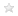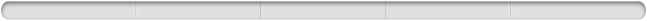This site uses cookies to deliver our services, improve performance, for analytics, and (if not signed in) for advertising. By using LibraryThing you acknowledge that you have read and understand our Terms of Service and Privacy Policy. Your use of the site and services is subject to these policies and terms.
Hide this

Click on a thumbnail to go to Google Books.

# Genetic Algorithms with Python (edition 2016)

## by Clinton Sheppard (Author)

 Members Reviews Popularity Average rating Conversations 3 None 3,596,851 None None Get a hands-on introduction to machine learning with genetic algorithms using Python. Step-by-step tutorials build your skills from Hello World! to optimizing one genetic algorithm with another, and finally genetic programming; thus preparing you to apply genetic algorithms to problems in your own field of expertise.Genetic algorithms are one of the tools you can use to apply machine learning to finding good, sometimes even optimal, solutions to problems that have billions of potential solutions. This book gives you experience making genetic algorithms work for you, using easy-to-follow example projects that you can fall back upon when learning to use other machine learning tools and techniques. Each chapter is a step-by-step tutorial that helps to build your skills at using genetic algorithms to solve problems using Python.Python is a high-level, low ceremony and powerful language whose code can be easily understood even by entry-level programmers. If you have experience with another programming language then you should have no difficulty learning Python by induction.ContentsChapter 1: Hello World! - Guess a password given the number of correct letters in the guess. Build a mutation engine.Chapter 2: One Max Problem - Produce an array of bits where all are 1s. Expands the engine to work with any type of gene.Chapter 3: Sorted Numbers - Produce a sorted integer array. Demonstrates handling multiple fitness goals and constraints between genes.Chapter 4: The 8 Queens Puzzle - Find safe Queen positions on an 8x8 board and then expand to NxN. Demonstrates the difference between phenotype and genotype.Chapter 5: Graph Coloring - Color a map of the United States using only 4 colors. Introduces standard data sets and working with files. Also introduces using rules to work with gene constraints.Chapter 6: Card Problem - More gene constraints. Introduces custom mutation, memetic algorithms, and the sum-of-difference technique. Also demonstrates a chromosome where the way a gene is used depends on its position in the gene array.Chapter 7: Knights Problem - Find the minimum number of knights required to attack all positions on a board. Introduces custom genes and gene-array creation. Also demonstrates local minimums and maximums.Chapter 8: Magic Squares - Find squares where all the rows, columns and both diagonals of an NxN matrix have the same sum. Introduces simulated annealing.Chapter 9: Knapsack Problem - Optimize the content of a container for one or more variables. Introduces branch and bound and variable length chromosomes.Chapter 10: Solving Linear Equations - Find the solutions to linear equations with 2, 3 and 4 unknowns. Branch and bound variation. Reinforces genotype flexibility.Chapter 11: Generating Sudoku - A guided exercise in generating Sudoku puzzles.Chapter 12: Traveling Salesman Problem (TSP) - Find the optimal route to visit cities. Introduces crossover and a pool of parents.Chapter 13: Approximating Pi - Find the two 10-bit numbers whose dividend is closest to Pi. Introduces using one genetic algorithm to tune another.Chapter 14: Equation Generation - Find the shortest equation that produces a specific result using addition, subtraction, multiplication, etc. Introduces symbolic genetic programming.Chapter 15: The Lawnmower Problem - Generate a series of instructions that cause a lawnmower to cut a field of grass. Genetic programming with control structures, objects and automatically defined functions (ADFs).Chapter 16: Logic Circuits - Generate circuits that behave like basic gates, gate combinations and finally a 2-bit adder. Introduces tree nodes and hill climbing.Chapter 17: Regular Expressions - Find regular expressions that match wanted strings. Introduces chromosome repair and growth control.Chapter 18: Tic-tac-toe - Create rules for playing the game.Source code: https://github.com/handcraftsman/GeneticAlgorithmsWithPython… (more)
 Member: realonedet Title: Genetic Algorithms with Python Authors: Clinton Sheppard (Author) Info: CreateSpace Independent Publishing Platform (2016), 532 pages Collections: Your library Rating:Tags: 2017-0102

#### Work Information

Genetic Algorithms with Python by Clinton Sheppard

2017-0102 (2)
NoneSign up for LibraryThing to find out whether you'll like this book.

No reviews
no reviews | add a review
For more help see the Common Knowledge help page.
Canonical titleOriginal titleAlternative titlesOriginal publication datePeople/CharactersImportant placesImportant eventsRelated moviesAwards and honorsEpigraphDedicationFirst wordsQuotationsLast wordsDisambiguation noticePublisher's editorsBlurbersOriginal languageCanonical DDC/MDSCanonical LCCReferences to this work on external resources.

##Wikipedia in English

None

Get a hands-on introduction to machine learning with genetic algorithms using Python. Step-by-step tutorials build your skills from Hello World! to optimizing one genetic algorithm with another, and finally genetic programming; thus preparing you to apply genetic algorithms to problems in your own field of expertise.Genetic algorithms are one of the tools you can use to apply machine learning to finding good, sometimes even optimal, solutions to problems that have billions of potential solutions. This book gives you experience making genetic algorithms work for you, using easy-to-follow example projects that you can fall back upon when learning to use other machine learning tools and techniques. Each chapter is a step-by-step tutorial that helps to build your skills at using genetic algorithms to solve problems using Python.Python is a high-level, low ceremony and powerful language whose code can be easily understood even by entry-level programmers. If you have experience with another programming language then you should have no difficulty learning Python by induction.ContentsChapter 1: Hello World! - Guess a password given the number of correct letters in the guess. Build a mutation engine.Chapter 2: One Max Problem - Produce an array of bits where all are 1s. Expands the engine to work with any type of gene.Chapter 3: Sorted Numbers - Produce a sorted integer array. Demonstrates handling multiple fitness goals and constraints between genes.Chapter 4: The 8 Queens Puzzle - Find safe Queen positions on an 8x8 board and then expand to NxN. Demonstrates the difference between phenotype and genotype.Chapter 5: Graph Coloring - Color a map of the United States using only 4 colors. Introduces standard data sets and working with files. Also introduces using rules to work with gene constraints.Chapter 6: Card Problem - More gene constraints. Introduces custom mutation, memetic algorithms, and the sum-of-difference technique. Also demonstrates a chromosome where the way a gene is used depends on its position in the gene array.Chapter 7: Knights Problem - Find the minimum number of knights required to attack all positions on a board. Introduces custom genes and gene-array creation. Also demonstrates local minimums and maximums.Chapter 8: Magic Squares - Find squares where all the rows, columns and both diagonals of an NxN matrix have the same sum. Introduces simulated annealing.Chapter 9: Knapsack Problem - Optimize the content of a container for one or more variables. Introduces branch and bound and variable length chromosomes.Chapter 10: Solving Linear Equations - Find the solutions to linear equations with 2, 3 and 4 unknowns. Branch and bound variation. Reinforces genotype flexibility.Chapter 11: Generating Sudoku - A guided exercise in generating Sudoku puzzles.Chapter 12: Traveling Salesman Problem (TSP) - Find the optimal route to visit cities. Introduces crossover and a pool of parents.Chapter 13: Approximating Pi - Find the two 10-bit numbers whose dividend is closest to Pi. Introduces using one genetic algorithm to tune another.Chapter 14: Equation Generation - Find the shortest equation that produces a specific result using addition, subtraction, multiplication, etc. Introduces symbolic genetic programming.Chapter 15: The Lawnmower Problem - Generate a series of instructions that cause a lawnmower to cut a field of grass. Genetic programming with control structures, objects and automatically defined functions (ADFs).Chapter 16: Logic Circuits - Generate circuits that behave like basic gates, gate combinations and finally a 2-bit adder. Introduces tree nodes and hill climbing.Chapter 17: Regular Expressions - Find regular expressions that match wanted strings. Introduces chromosome repair and growth control.Chapter 18: Tic-tac-toe - Create rules for playing the game.Source code: https://github.com/handcraftsman/GeneticAlgorithmsWithPython

No library descriptions found.

Book descriptionHaiku summary